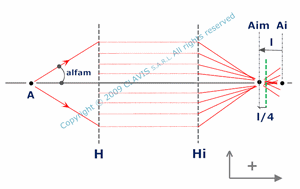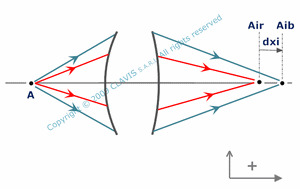and ortutorials

# aberrations

Geometric aberrationsFor optical systems which are not rigorously stigmatic and when Gauss conditions are not respected, aberrations may appear. It is then no more possible to consider that the optical system transform light beam coming from an object point into a beam converging to an image point. Whatever the observation plane position, the emerging light rays draw on it a spot with non null dimensions. It is then said that the optical system has aberration. There are different kinds of aberrations, depending on the distance of the object to the optical axis ( axis around which the optical system is assumed to have a symmetry of revolution ) and/or the angle of incident light rays with the optical axis ( incident light rays angle ).

Spherical aberrationAmong all the geometric aberrations, one occurs for object points on the optical axis. It is called the spherical aberration. It appears for large enough incident light rays angle. It depends on object position, aperture, geometry/orientation of the optical surfaces, refraction indexes of the different media and distances between consecutive optical surfaces. Because of the symmetry of revolution, the optical system transforms incident light rays with the same angle and coming from the same object point A on the axis into rays focusing on a same image point on the axis. For angles close to zero, this image point is the paraxial image Ai of the object point. As the image position changes with incident rays angle, approached stigmatism is no more achieved. Whatever the position of the observation plane, a spot with non null dimensions and with a circular shape is observed. Aim being the conjugate of A for marginal rays ( rays with the maximum angle alfam ), the distance l between Ai and Aim is called longitudinal spherical aberration. The spot diameter dy in the paraxial image plane ( including Ai ) is called transversal spherical aberration.
The angle alfam is defined by the aperture diameter and the distance from the object to this aperture. In the calculation pages, it is assumed that the aperture is on the first optical surface of the optical component or system considered and not necessarily in the plane perpendicular to the optical axis and containing the vertex of the first surface.

Generally, spot diameter is minimum in a plane between Ai and Aim located at around l/4 from Aim.

Spherical lenses exhibit spherical aberration. Other commonly used components like spherical mirrors and plane parallel plates also have spherical aberration. Consequently optical systems made of spherical lenses and/or spherical mirrors and/or parallel plane plates may generate spherical aberration.

There are solutions for minimizing spherical aberration like, for example, using aspherical facets for lens or Schmidt plate for a spherical mirror when object is at infinity...

Chromatic aberrationAnother type of aberration exists for optical systems including some refractive components. Unlike geometric aberrations, chromatic aberration occurs also in Gauss conditions for objects close to the axis and light rays direction close to axis direction. Unlike reflective components, refractive components generally contain a dispersive material ( which refraction index depends on the wavelength ). The same incident ray will be refracted in different directions at the component interface depending on the wavelength. Consequently, the image position of an object point by the optical system will be different depending on the wavelength. Practically, if the object point is illuminated by a polychromatic light source, it has as many conjugated points as wavelengths of the light source, and whatever the position of the observation plane, emerging rays draw an aberration spot with non null dimensions.Depending on object position, different types of chromatic aberrations occur. For an object A on the optical axis and a given light source spectrum, the axial chromatism is given by the algebric distance ( dxi ) from Air to Aib, Air and Aib being the conjugated points respectively for the largest and the smallest wavelengths of the spectrum. Principal axial chromatism applies for object at infinity. It is then characterized by the algebric distance ( dfi ) from Fir to Fib, Fir and Fib being the back focal points respectively for the largest and the smallest wavelengths of the spectrum.Transverse chromatism occurs for objects out of the optical axis. It's mainly characterized by the fact that the magnification depends on wavelength. Part of magnification change is caused by the longitudinal chromatism and is called height chromatism (dyi). For an object point B out of the axis the height chromatism ( dyi ) is given by the algebric height difference between Br and Bb, Br and Bb being the paraxial images of B respectively for the largest and the smallest wavelengths of the spectrum.In the visible, largest and smallest wavelength currently used are 656,27 nm ( red hydrogen line ) and 486,13 nm ( blue hydrogen line ). The dispersion of materials is commonly characterized by the constringence. Constingence is calculated using refraction indexes at 486,13 nm (blue), 587,56 nm ( yellow helium line ) and 656,27 nm ( red ). The higher is the constringence, the less dispersive is the material. In a standard way, both transverse and axial chromatism of a thin lens depend on constringence ( nu ), effective focal length ( fi ) and object position ( x ). Height chromatism (dyi ) depends also on object's height ( y ). Principal axial chromatism is a function of fi and nu.By using two dedicated thin lenses ( L1and L2 ) placed side by side, it is possible to make Fib ( back focal point of the two lenses system at 486,13 nm ) merge with Fir ( back focal point of the two lenses system at 656,28 nm ). It is then said that principal axial chromatism is compensated and the system is an achromat for an object point at infinity. Generally, one of the lens is converging and the other is diverging. L1 and L2 are made in different materials with different constringence nu1 and nu2. Their effective focal lengths ( fi1 and fi2 ) can be calculated as a function of nu1, nu2 and fi, fi being effective focal length desired for the achromat. More generally, chromatism can be calculated whatever small and large wavelength chosen ( not necessarily those of the standard spectrum mentioned above ).In the general case, height and axial chromatism of a spherical lens are functions of central thickness ( e ), facets radii ( R1 and R2 ), and refraction indexes ( ns and nl ) for the small and large wavelengths considered. Height chromatism depends also on object's height ( y ).

References

"Optique Fondements et applications" - 2004 - author : José-Philippe Perez.

"Optique géométrique Imagerie et instruments" - 2007 - author : Bernard Balland.

"Optique géométrique paraxiale" - Institut d'optique théorique et appliquée - 1985 - author : Michel Cagnet.

"Formation des images Aberrations" - Institut d'optique théorique et appliquée - 1985 - author : Michel Cagnet.